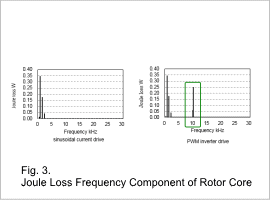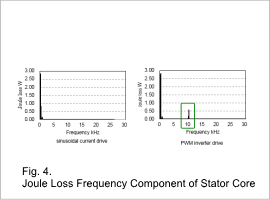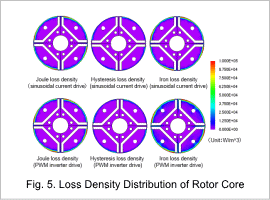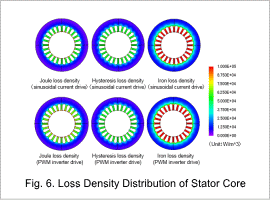## [JAC090] Analysis of the Effect of PWM on the Iron Loss of an IPM Motor

Remember me

*It is different from the service for JMAG WEB MEMBER (free member). Please be careful.
About authentication ID for JMAG website

### Overview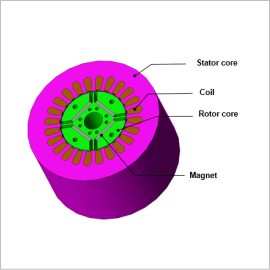Current vector controls are generally used in interior permanent magnet synchronous motors (hereinafter referred to as IPMs), and among them PWM inverters are widely utilized to create a command current. It is vital to get a good understanding of iron losses in order to raise the efficiency of an IPM motor. However, iron losses increase when power is converted by the PWM inverter because the carrier harmonic created by the PWM becomes superimposed on the current and the magnetic flux density waveform in the IPM motor’s core.
There are three methods to gain an understanding of iron loss that accounts for PWM carrier harmonics: There is the method of linking a control / circuit simulator that contains the PWM inverter with a magnetic field analysis by inputting the current waveform obtained from the simulation into the analysis, the method of inputting the actual measurements of a current into a magnetic field analysis, and the method of inputting the estimated harmonic current.
This Application Note demonstrates a case study where the effects of a carrier harmonic against an IPM motor’s iron loss is confirmed using estimated harmonic current waveform as input.

### Iron Loss

A comparison of the hysteresis losses, and joule losses when the IPM motor is driven by a PWM inverter and a sinusoidal current are shown in fig 1 and Fig. 2.
The iron losses in both the rotor core and the stator core increase when the IPM motor is run using a PWM inverter. The joule losses increase the most severely, increasing 70% in the rotor core, and 20% in the stator core.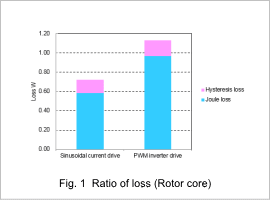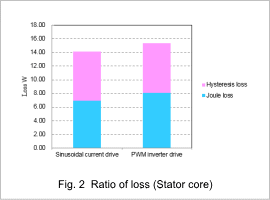### Joule Loss/ Joule Loss Density Distribution

The joule loss frequency components are shown in Fig. 3 and Fig. 4, and the joule loss density distribution is shown in Fig. 5 and Fig. 6.
The fundamental frequencies (360 Hz in the rotor core and 60 Hz in the stator core) and harmonic components have almost the same amounts of iron loss in the rotor core and stator core whether a sinusoidal current drive or a PWM inverter drive is used. However, iron loss is only produced during PWM inverter drive for the PWM‘s fundamental frequency (10 kHz). (These values are indicated inside the green square in the Fig. 3 and Fig. 4.)
The increased joule losses when the motor is run using a PWM inverter are caused by the carrier harmonic.
The effects of a carrier harmonic need to be evaluated to analysis the characteristics of an IPM motor in detail.